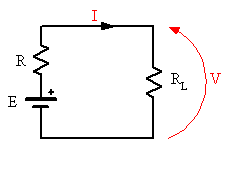# Change in voltage due to additional source

• Queequeg
In summary, when an identical voltage source is added to the original in parallel, the effective internal resistance is halved while the voltage across the load remains unchanged. When added in series, the effective resistance is doubled and the voltage across the load is doubled as well. The effect on the load voltage will depend on the type of load, but it can be assumed that it is resistive.

## Homework Statement

A voltage source connected to a circuit produces a voltage V, current I and has internal resistance R. What is the new voltage if an identical voltage source is added to the original:

a) in parallel
b) in series

V=IR[/B]

## The Attempt at a Solution

a. For a voltage source in parallel, the equivalent resistance is R/2. Each source provides a current I so the total current is I+I=2I. Therefore the new voltage is V_n=(2I)(R/2)=IR=V unchanged

b. The equivalent resistance is 2R and the current is I because they are in series, so the new voltage is V_n=I(2R)=2V doubled.

Did you have any further questions?

Queequeg said:

## Homework Statement

A voltage source connected to a circuit produces a voltage V, current I and has internal resistance R. What is the new voltage if an identical voltage source is added to the original:

a) in parallel
b) in series

V=IR[/B]

## The Attempt at a Solution

a. For a voltage source in parallel, the equivalent resistance is R/2. Each source provides a current I so the total current is I+I=2I. Therefore the new voltage is V_n=(2I)(R/2)=IR=V unchanged

b. The equivalent resistance is 2R and the current is I because they are in series, so the new voltage is V_n=I(2R)=2V doubled.
What is the effect of the circuit to which the voltage supplies are connected? Presumably the voltage mentioned is meant to be the voltage across that load?When you connect an identical source in parallel with the first, I agree that the effective internal resistance is halved while the effective cell voltage remains the same. But how will that effect the voltage V that is measured across the load resistance?